# 【OpenCV】数字图像的表示 | 图像IO操作接口 | 图像混合操作

## Ⅰ. 数字图像的表示

### 0x00 位数

•  0：代表最黑
•  1：表示最白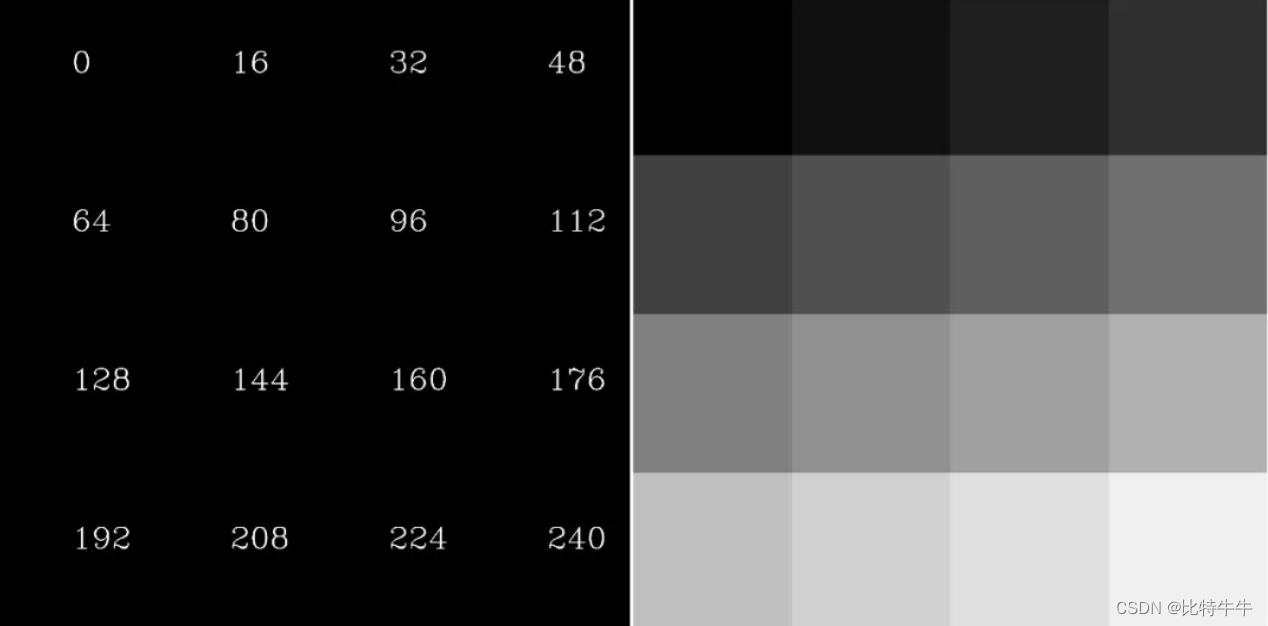### 0x01 二值图像

“0”代表黑色，“1”代白色。由于每一像素（矩阵中每一元素）。

图像与索引图像一样都可以用来表示彩色图像。

分别表示图像的行列数，

3 个 的二维矩阵分别表示各个像素的  三个颜色分量。

图像的数据类型一般为 8 位无符号整形，通常用于表示和存放真彩色图像。

• 像素点取值范围：

## Ⅱ. OpenCV 基本操作

### 0x00 图像 I/O 操作的 API

 cv2.imread()     # 读取图像

* 注： 可以使用 1、0 或者 -1 来替代上面三个标志

cv2.imshow()：     #显示图像

📌 注意：在调用显示图像的 API 后，要调用 cv.waitKey() 给图像绘制留下时间，否则窗口会出现无响应情况，并且图像无法显示出来。

cv2.imwrite():        #保存图像

•  cv2.line(): 绘制直线
•  cv2.circle(): 绘制圆形
•  cv2.rectangle(): 绘制矩形
•  cv2.putText(): 在图像上添加文字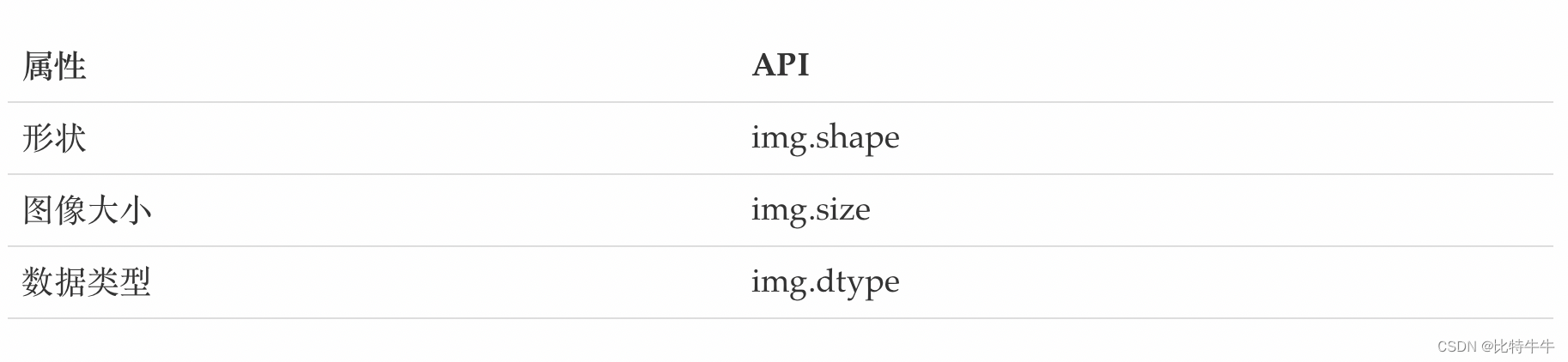拆分通道：

cv.split()

通道合并：

cv.merge()

色彩空间的改变：

cv.cvtColor(input_image，flag)

💎 举例：

•  数据读取 —— 图像：
image = cv2.imread("sunshine.jpg")
gray_image = cv2.cvtColor(image, cv2.COLOR_BGR2GRAY)
cv2.imshow("Over theClouds - gray", gray_image)
cv2.waitKey(0)#等待时间，毫秒级，0表示按任意键终止• 截取部分图像数据
img = cv2.imread("sunshine.jpg")#读取图片
sunshine = img[0:80, 10:200]#切片
cv2.imshow("sunshine", sunshine)
cv2.waitKey(0)#等待时间，毫秒级，0表示按任意键终止
• 颜色通道提取

img[:, :, 0] = 0
img[:, :, 1] = 0img[:, :, 0] = 0
img[:, :, 2] = 0top_size, bottom_size, left_size, right_size = (200, 200, 200, 200)
replicate = cv2.copyMakeBorder(img, top_size, bottom_size, left_size, right_size, borderType=cv2.BORDER_REPLICATE)
reflect = cv2.copyMakeBorder(img, top_size, bottom_size, left_size, right_size, borderType=cv2.BORDER_REFLECT)
reflect_101= cv2.copyMakeBorder(img, top_size, bottom_size, left_size, right_size, borderType=cv2.BORDER_REFLECT_101)
wrap = cv2.copyMakeBorder(img, top_size, bottom_size, left_size, right_size, borderType=cv2.BORDER_WRAP)
constant = cv2.copyMakeBorder(img, top_size, bottom_size, left_size, right_size, borderType=cv2.BORDER_CONSTANT, value=0)#zero padding
plt.subplot(231), plt.imshow(sunshine), plt.title('ORIGINAL')
plt.subplot(232), plt.imshow(replicate), plt.title('REPLICATE')
plt.subplot(233), plt.imshow(reflect), plt.title('REFLECT')
plt.subplot(234), plt.imshow(reflect_101), plt.title('REFLECT_101')
plt.subplot(235), plt.imshow(wrap), plt.title('WRAP')
plt.subplot(236), plt.imshow(constant), plt.title('CONSTANT')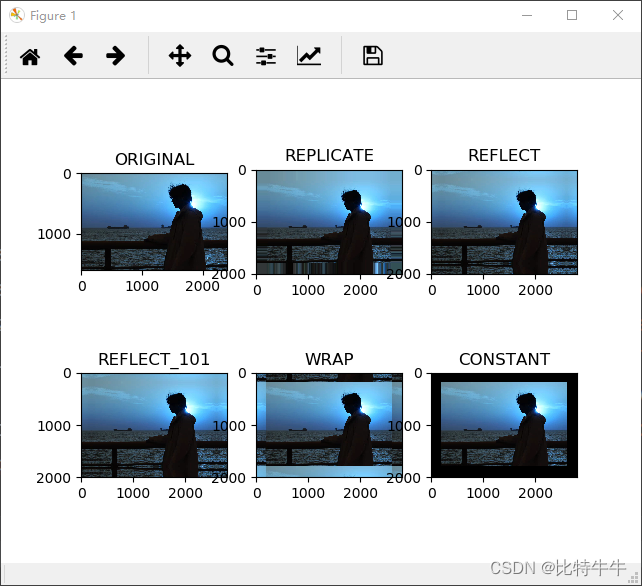• BORDER_REPLICATE：复制法，复制最边缘像素
• BORDER_REFLECT： 反射法，对感兴趣的图像中的像素在两边进行复制
• BORDER_REFLECT_101：反射法，一最边缘的像素为轴
• BORDER_WRAP：外包装法
• BORDER_CONSTANT：常量法，常数值填充

### 0x01  算数操作

📌 注意：

加法和 Numpy 加法之间存在差异，的加法是饱和操作，而 Numpy 添加是模运算。

💬 参考以下代码：

>>> x = np.uint8()
>>> y = np.uint8()
>>> print( cv.add(x,y) ) # 250+10 = 260 => 255
[]
>>> print( x+y )          # 250+10 = 260 % 256 = 4


💎 示例：

img_sunshine = cv2.resize(img_sunshine, (500, 250))
img_cloud = cv2.resize(img_cloud, (500, 250))

res = cv2.addWeighted(img_sunshine, 0.4, img_cloud, 0.6, 0)
plt.imshow(res)
plt.show()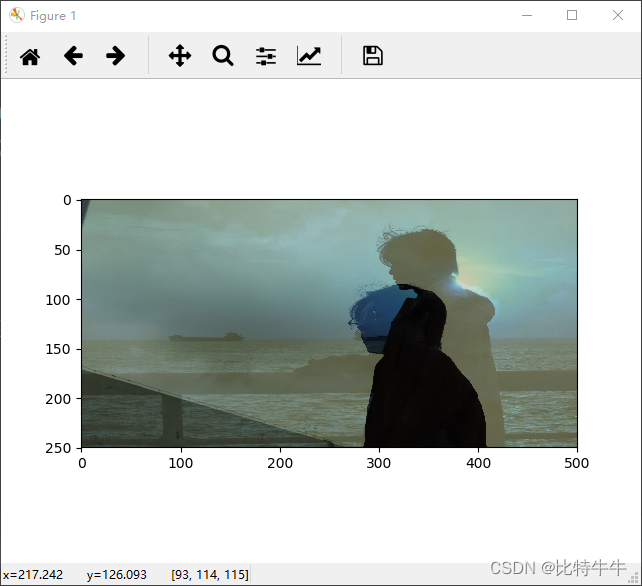res2 = cv2.resize(img_sunshine, (0, 0), fx=3, fy=1)
plt.imshow(res2)
plt.show()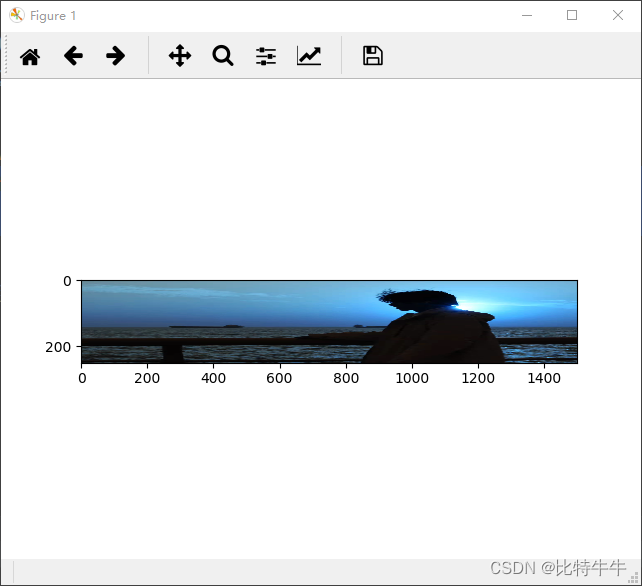1. 图像加法：将两幅图像加载一起

2. 图像的混合：将两幅图像按照不同的比例进行混合

(0)